# FINDITEM formulaHi all,

I have a list named "TAT Hours" and a line item "TOTAL HOURS" that is in number format.

I used FINDITEM formula to get the number of hours (TAT Hours) in the list, here is the formula:

FINDITEM(TAT Hours, TEXT(IF TOTAL HOURS <= VALUE(NAME(TAT Type.Min Hours[LOOKUP: 'TAT Type1'])) THEN VALUE(NAME(TAT Type.Min Hours[LOOKUP: 'TAT Type1'])) ELSE IF TOTAL HOURS > VALUE(NAME(TAT Type.Max Hours[LOOKUP: 'TAT Type1'])) THEN VALUE(NAME(TAT Type.Max Hours[LOOKUP: 'TAT Type1'])) ELSE TOTAL HOURS))

It gives me the correct result, which is the corresponding "TAT Hours", but I noticed that if I will input any number in line item TOTAL HOURS that ends in zero (0) it will not give any result, just blank. But if I will input any other number that is not ending with zero, it gives back the correct result.

I attached a screenshot, wherein the first line item gives the correct result but the next item does not (since it ends with zero)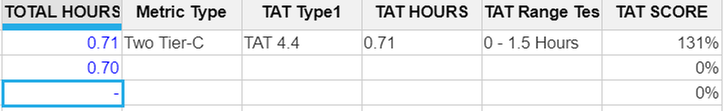Thank you,

Sarah

Tagged:

•Silly question:

Any reason why you can't set the code for 0.10 to 0.1, 0.20 to 0.2 etc.?

That makes it easy!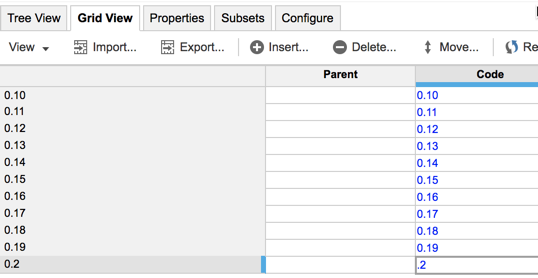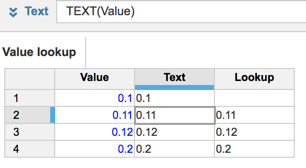David

•Alternatively, this might work

Check for the length of the "number" and create the text string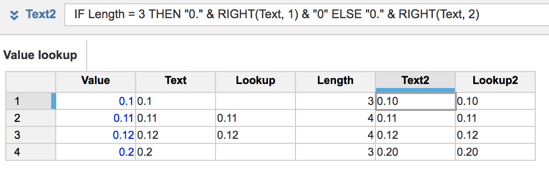David

•Brilliant

The one thing I really stress to everyone is take that step back and look at the problem differently and laterally and logically  All too often we go too deep too soon and miss the obvious.  That is not a criticism, but I think modellers tend to think Anaplan must be complicated because of the nature of multi-dimensionality.  Anaplan is designed to be simple and often the best solutions are the most obvious.

I've started quoting Tim Peters:

Simple is better than complex, complex is better than complicated.

David

•Firstly I would definitely split that formula up.

2. It will be much more efficient

3. You have the same formula expressions repeated multiple time

• VALUE(NAME(TAT Type.Min Hours[LOOKUP: 'TAT Type1']
• VALUE(NAME(TAT Type.Max Hours[LOOKUP: 'TAT Type1']

I suspect the problem is that the value of 0.70 is resolving to 0.7.  If the name or code code of the TAT Hours is 0.7 it will not find it.  try changing the name and/code to 0.70

But definitely split up that formula!

David

•Hi, thank you @DavidSmith for the response. I also thought of that, that Anaplan might read it as 0.7 instead of 0.70 but the TAT Hours list looks like this (list name and code in 2 decimal places, with zero in the end):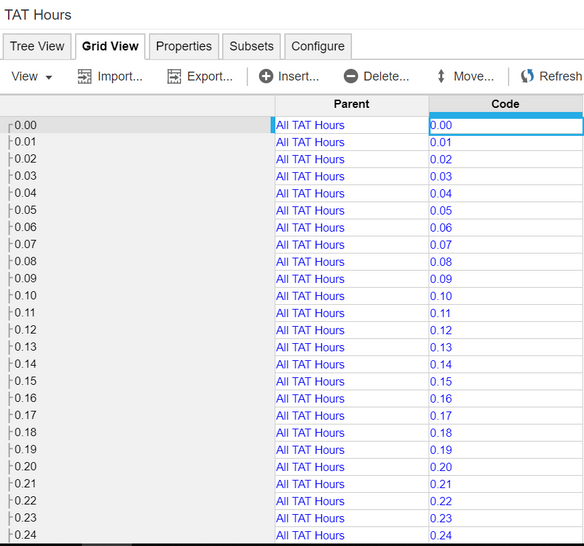Also now, I tried to look up using number rounded up in 2 decimal place (used Round(Total Hours, 2) formula for it to match the decimal places in the TAT Hours list) but the same problem goes.

Hope you can help me with this, THANK YOU.

•This silly question solved my query. I am thinking way too far than how simple the solution should be. It took one silly question for me to think 🙂 THANK YOU again for this @DavidSmith, my module now calculates all the hours I need 🙂